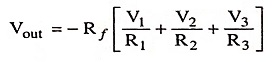## Linear Applications of Op-Amps Interview Questions and Answers:

1. Give the examples of linear circuits.

Ans. Adder, subtractor, differentiator, integrator, voltage-to-current converter, current-to-voltage converter, instrumentation amplifier fall under the category of linear circuits.

2. What is an adder or summing amplifier?

Ans. Adder or summing amplifier is a circuit that provides an output voltage proportional to or equal to the algebraic sum of two or more input voltages multiplied by a constant gain factor.

3. What is scaling amplifier?

Ans. If the input voltage is amplified by a different factor i.e., weighted differently at the output, the circuit becomes a scaling or weighted amplifier. The output voltage is given by Eq.where R1 # R2 # R3.

4. What is another name for voltage-to-current converter?

Ans. The another name for voltage-to-current converter is transcon­ductance amplifier.

5. What is current-to-voltage converter?

Ans. A device that produces a voltage proportional to input signal current is called a current-to-voltage converter.

6. What is difference between positive scalar and voltage-to-­current converter?

Ans. The circuit of voltage-to-current converter is similar to that of a positive scalar except that feedback resistor Rf is replaced by load resistor RL.

7.What is dc amplifier?

Ans. DC amplifier is a device in which output signal changes in response to changes in its dc input levels.

8. How is accuracy of dc amplifiers improved?

Ans. The accuracy of dc amplifiers is improved by using an offset null circuitry of the op-amp. This reduces the output offset voltage to zero.

9. Name any two instrumentation amplifiers?

Ans. The two instrumentation amplifiers may be

1. Instrumentation amplifier using transducer bridge.
2. Instrumentation amplifier using temperature controller.

10. Why a potentiometer is provided in an instrumentation amplifier?

Ans. A potentiometer is provided, in an instrumentation amplifier, to permit adjustment of the scale factor of the circuit.

11. What is an integrator?

Ans. An integrator is a circuit that performs a mathematical operation called integration.

12. What are the applications of integrators?

Ans. Integrators are widely used in ramp or sweep generators, filters, analog computers etc.

13. Why integrators are preferred over differentiators?

Ans. Op-amp is mostly used as an integrator rather as a differentiator, because in differentiator at high frequency, gain is high and so high frequency noise is also amplified which absolutely abstract the differentiated signal.

14. What do you mean by differentiator?

Ans. A differentiator or differentiation amplifier is a circuit that performs the mathematical operation of differentiation i.e., the output voltage is proportional to the rate of change of the input voltage. It is an inverse mathematical operation to that of integrator.

A differentiator is rarely used in analog computers as it tends to amplify (the output voltage magnitude being proportional to the frequency of the input signal) noise drift and other  unwanted disturbances in the system.

Scroll to Top